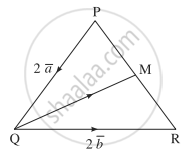# In the triangle PQR, PQ2aQR2bPQ¯=2a¯,QR¯=2b¯. The midpoint of PR is M. Find the following vectors in terms of aa¯ and bb¯: (i) PRPR¯ (ii) PMPM¯ (iii) QMQM¯. - Mathematics and Statistics

Sum

In the triangle PQR, bar"PQ" = bar"2a", bar"QR" = bar"2b". The midpoint of PR is M. Find the following vectors in terms of bar"a" and bar"b":

(i) bar"PR" (ii) bar"PM" (iii) bar"QM".

#### Solutionbar"PQ" = bar"2a" , bar"QR" = bar"2b"

(i) bar"PR" = bar"PQ" + bar"QR"

= 2bar"a" + 2barb

(ii) ∵ M is the midpoint of PR

∴ bar"PM" = 1/2bar"PR" = 1/2[2bar"a" + 2bar"b"]

= bar"a" + bar"b"

(iii) bar"RM" = 1/2(bar"RP") = - 1/2 bar"PR" = - 1/2(2bar"a" + 2bar"b")

= - bar"a" - bar"b"

∴ bar"QM" = bar"QR" + bar"RM"

= 2bar"b" - bar"a" - bar"b"

= bar"b" - bar"a"

[Note: point (i) answer in the textbook is incorrect.]

Concept: Vectors and Their Types
Is there an error in this question or solution?